### Common Collector Configuration: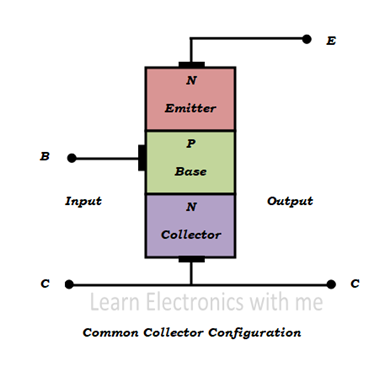Common collector configuration

Two terminals are needed for input and two terminals for output. Transistors have three terminals, so one terminal have to be taken as common terminal for both input and output.

In common collector configuration collector terminal is taken as common. So input is applied between base and the collector terminals and output is taken from emitter and collector terminals.

The common collector configuration is also called emitter follower or voltage follower because the output emitter voltage always follows the base input voltage.

For example the base emitter voltage is 0.7v and if the input is 5V then the output is 4.3V. Output voltage is always near the input voltage. This configuration is widely used as a buffer and it is also called as voltage buffer.

### Circuit diagram of NPN and PNP configuration: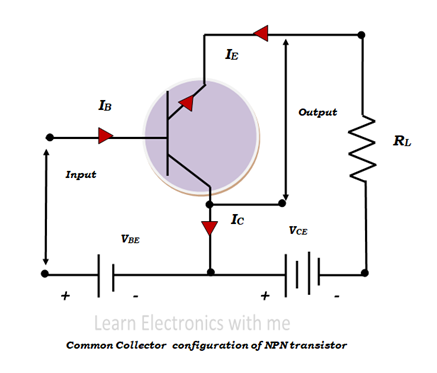Common collector configuration of NPN transistor

Input is applied between the base and the collector terminals. Input current that is the base current is denoted as IB and the input voltage that is the base emitter voltage is denoted as VBE. Collector terminal which is taken as common is grounded.

The output current is the emitter current, it is denoted as IE and the output voltage is emitter collector voltage and it is denoted as VCE. The output emitter current is the summation of the base and collector current. Emitter junction is forward biased and collector junction is reverse biased.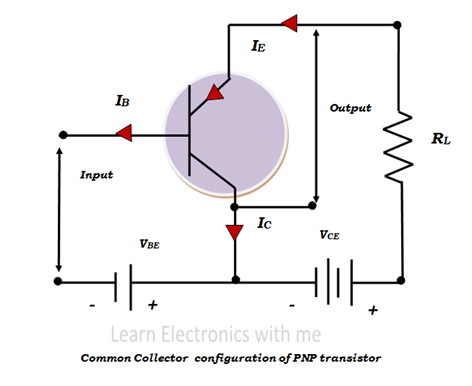Common collector configuration of PNP transistor

Common collector configuration have high input configuration and low output impedance. It has low voltage gain, high current gain and the power gain is medium. This configuration is mostly used for impedance matching, that is high impedance source is used to drive low impedance load.

### Input Characteristics of Common Collector Configuration: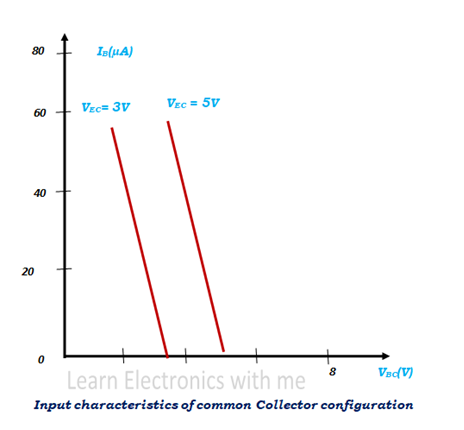Input characteristics of common collector configuration

Input characteristics are the relationship between the input current and input voltage keeping output voltage constant. Here input current is IB and input voltage VBE and output voltage is VCE.

The output voltage VCE initially kept at 3V and kept constant. The input voltage VBE is increased from zero gradually and the corresponding input current IB is noted.

Then the output voltage which is kept constant is increased, let’s say 5V and kept constant and output voltage is gradually increased and input current is noted. Graph is drawn with all the values noted.

### Output Characteristics of Common Collector Configuration: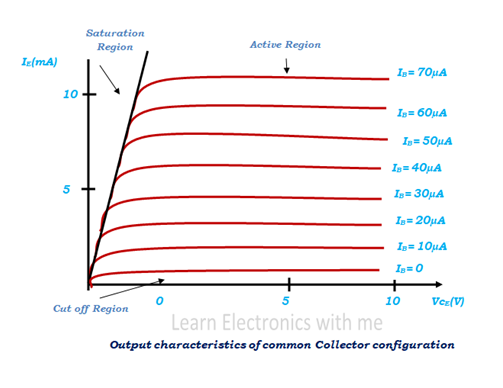Output characteristics of common collector configuration

Output characteristics are the relationship between the output current and output voltage keeping input current constant. Here output current is IE and output voltage is VCE and the input current is IB.

Initially the input current IB is kept at zero and kept constant. Slowly input current IB is increased like 10µA,20µA and kept constant and the output voltage VCE is increased gradually from zero and the corresponding output current IE is noted.

When the input current is zero no current flows in the transistor and it is called cut off region. When the input current is very high the current through the transistor is also very high and the transistor will be in saturation region.

The region where there is a change in the output current for the change in output voltage is the active region. Here the active region almost looks flat.